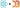# Challenge

Kiran has a problem. He's working on a project and doesn't know how. Let's help

# My Solution

Kiran wants to build a "circle with arcs" chart, but he's having trouble. He asked for help so here we are :)I livecoded this one from the Paris airport so there's no sound in the video. I was too shy to narrate my actions in the middle of a busy Starbucks. Maybe next time.

Anyway, to build an arc circle like this, we can take many cues from how you would build a piechart. Arcs are still arcs: They're round, have an inner and outer radius, and represent a datapoint. We can layer them on top of each other with a band scale feeding into radiuses.

Like this 👇

## First you need a dataset

We fake the dataset because Kiran didn't provide one.

`// 5 percentages represent our datasetconst data = d3.range(5).map(_ => ({  name: Faker.hacker.verb(),  percentage: 75 * Math.random(),}));`

5 datapoints, fake name with faker, and a random chunk out of 75%. Tried going full 100 at first and it didn't look great at all.

## Then you need a parent component

```const CircleArcs = ({ data, maxR }) => {  const rScale = d3    .scaleBand()    .paddingInner(0.4)    .paddingOuter(1)    .domain(d3.range(data.length))    .range([0, maxR]);.css-13aqjzy{display:inline-block;}
return (    <g>      <Circle cx={0} cy={0} r={maxR} />      {data.map((d, i) => (        <Arc d={d} r={rScale(i)} width={rScale.bandwidth()} key={i} />      ))}    </g>  );};```

A functional component will do. Create a band scale for the radiuses. Those cut up a given space into equal bands and let you define padding. Same scale you'd use for a barchart to position the bars.

The band scale is ordinal so our domain has to match the number of inputs, `d3.range` takes care of that. For our dataset that sets the domain to `[0,1,2,3,4]`.

Scale range goes from zero to max radius.

Render a `<Circle>` which is a styled circle component, loop through the data and render an `<Arc>` component for each entry. The arc takes data in the `d` prop, call `rScale` to get the radius, and use `rScale.bandwidth()` to define the width. Band scales calculate optimal widths on their own.

We can use index for keys because we know arcs will never re-order.

## The parent component needs arcs

That's what it's rendering. They look like this

```const Arc = ({ d, r, width }) => {  const arc = d3    .arc()    .innerRadius(r)    .outerRadius(r + width)    .startAngle(0)    .endAngle((d.percentage / 100) * (Math.PI * 2));
return (    <g>      <Label y={-r} x={-10}>        {d.name}      </Label>      <ArcPath d={arc()} />    </g>  );};```

A D3 arc generator defines the path shape of our arcs. Inner radius comes from the `r` prop, outer radius is `r+width`. Unlike a traditional pie chart, every arc starts at angle zero.

The end angle makes our arcs communicate their value. A percentage of full circle.

Each arc also comes with a label at its start. We position those at the beginning of the arc using the `x` and `y` props. Setting their anchor point as `end` automatically makes them end at that point.

```const ArcPath = styled.path`  fill: white;`;
const Label = styled.text`  fill: white;  text-anchor: end;`;```

Styled components work great for setting pretty much any SVG prop 👌

And the result is a circle arc chart thing. Wonderful.

Previous:
Real-time WebGL map of all airplanes in the world
Next:
Which emails sparked joy – an animated timeline
Created bySwizecwith ❤️